# Unity Shader 基础教程

### 第二部分：渲染管道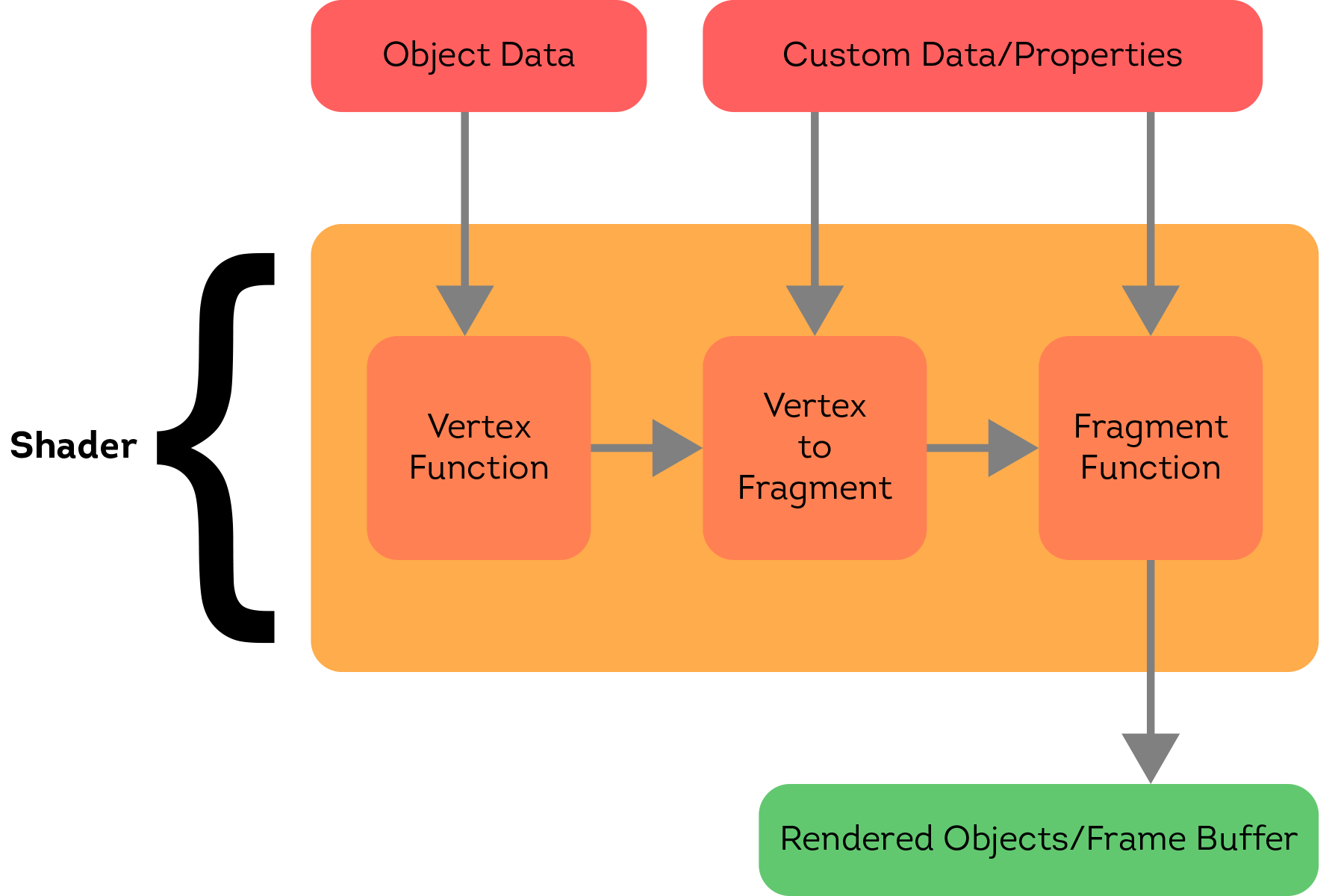### 第三部分：Scene 配置

• Bowl Model(碗模型)
• Noise Texture(噪声纹理)
• Bowl texture(碗模型纹理)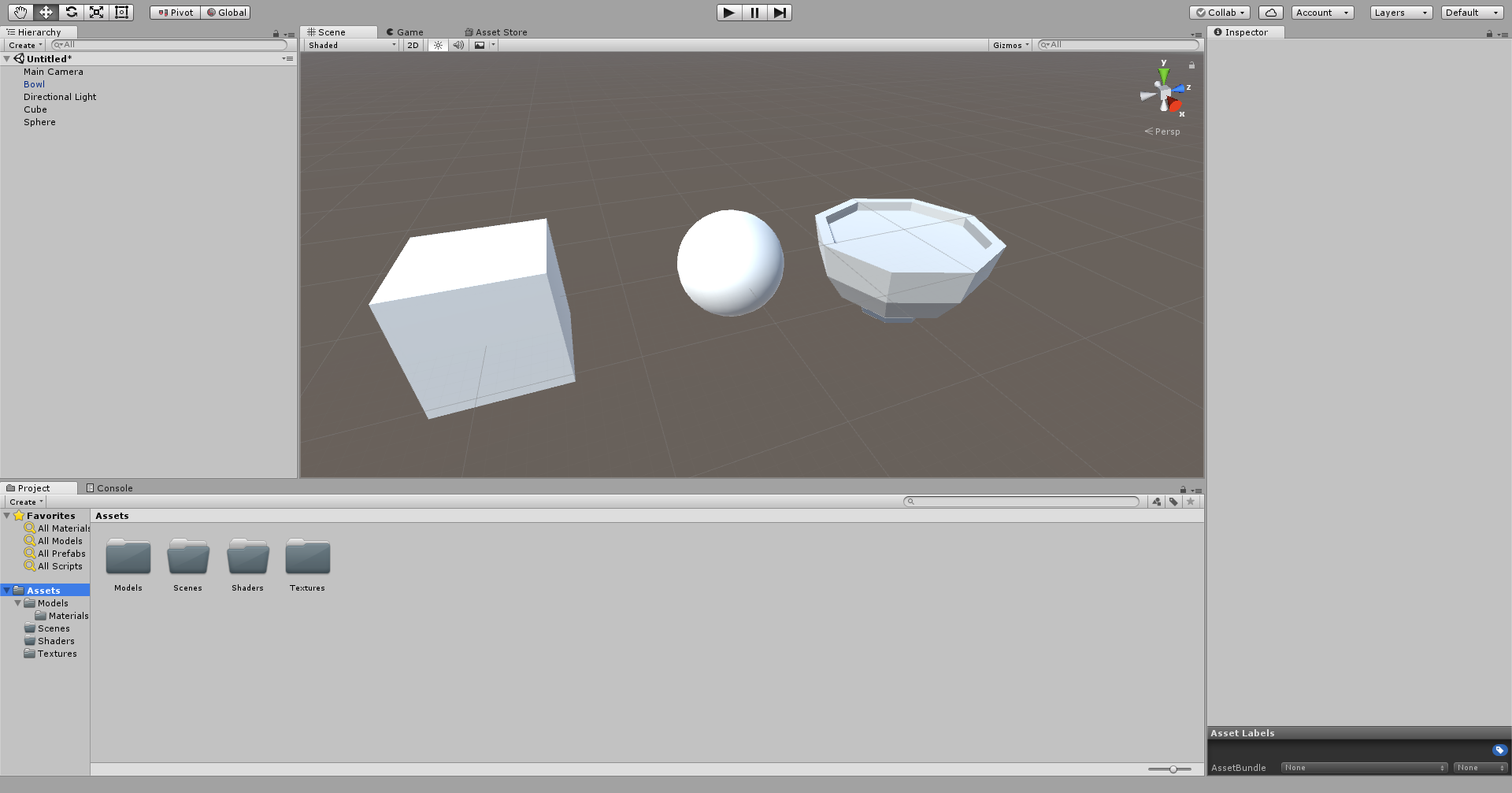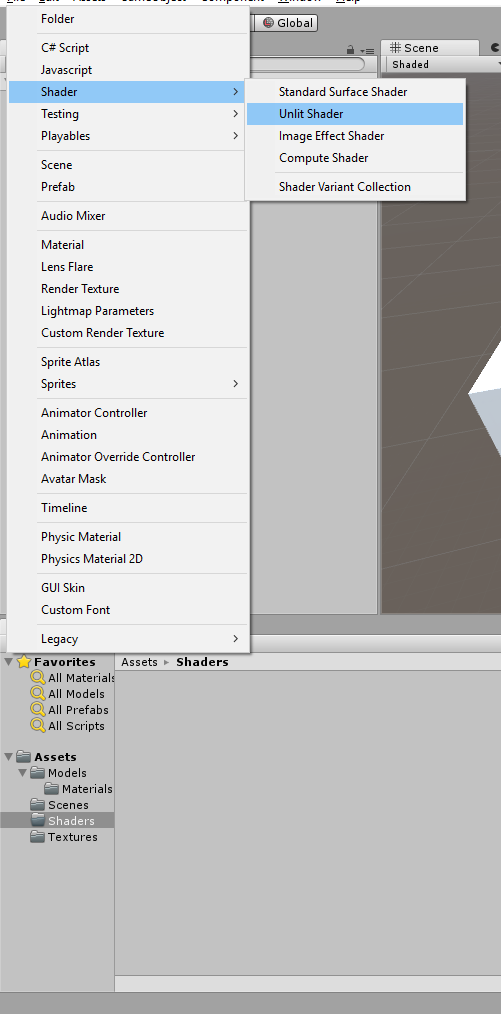Note:一个“Material”在Unity中仅仅是着色器的一个实例，它仅保存自定义数据/属性的值。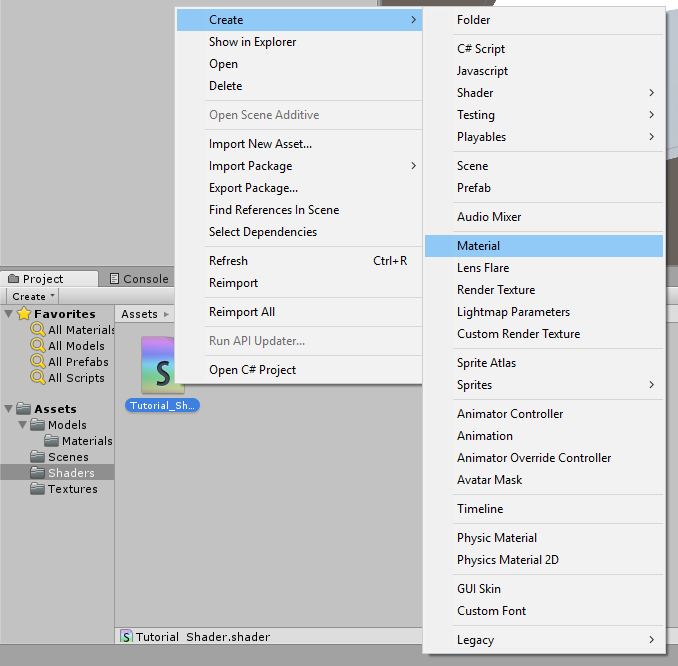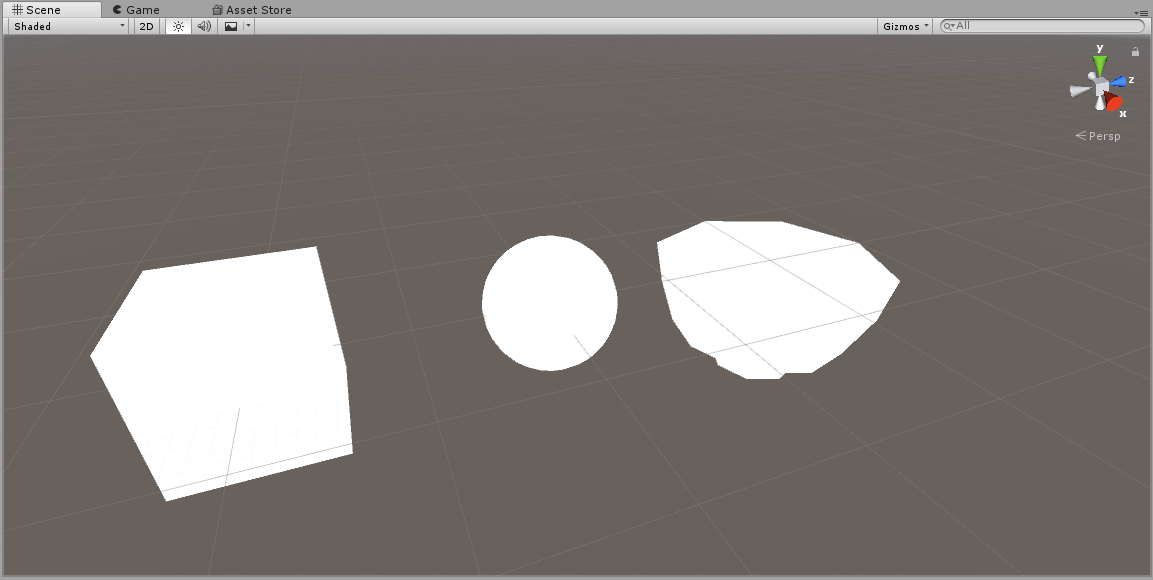Shader "Unlit/Tutorial_Shader"{
...
}

Shader "A/B/C/D/E_Shader"{
...
}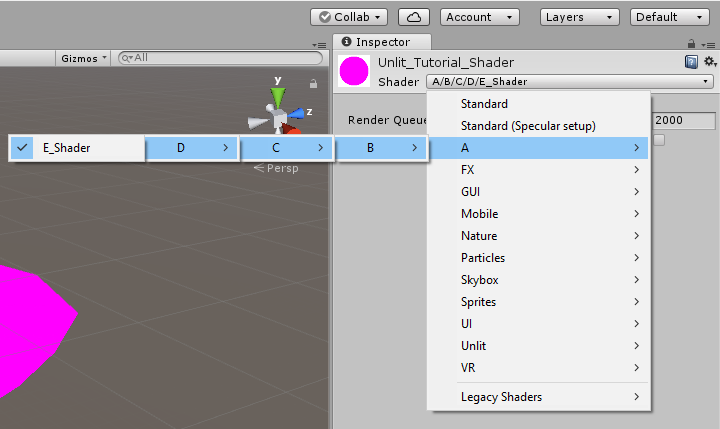Shader "Unlit/Tutorial_Shader"{
Properties{
...
}
}

Shader "Unlit/Tutorial_Shader"{
Properties{
...
}
...
}
}

pass语句块：

Shader "Unlit/Tutorial_Shader"{
Properties{
...
}
Pass{
...
}
}
}

Shader "Unlit/Tutorial_Shader"{
Properties{

}
pass{
CGPROGRAM
...
ENDCG
}
}
}

CGPROGRAM
#pragma vertex vertexFunction
#pragma fragment fragmentFunction
ENDCG

CGPROGRAM
#pragma vertex vertexFunction
#pragma fragment fragmentFunction

void vertexFunction(){

}
void fragmentFunction(){

}
ENDCG

CGPROGRAM
#pragma vertex vertexFunction
#pragma fragment fragmentFunction

#include "UnityCG.cginc"

struct a2v{

};
void vertexFunction(a2v v){

}
void fragmentFunction(){

}
ENDCG

[type] [name] :[semantic]

flot4 vertex:POSITION;

struct a2v{
float4 vertex:POSITION;
float2 uv:TEXCOORD0;
}

CGPROGRAM
#pragma vertex vertexFunction
#pragma fragment fragmentFunction
#include "UnityCG.cginc"

struct a2v{
float4 vertex:POSITION;
float2 uv:TEXCOORD0;
};
struct v2f{

};
v2f vertexFunction(a2v v){
v2f o;
return o;
}
void fragmentFunction(){

}

ENDCG

struct v2f{
float4 position:SV_POSITION;
float2 uv:TEXCOORD0;
}

fixed4 fragmentFunction(v2f i){

}

fixed4 fragmentFunction(v2f i):SV_TARGET{

}

Shader "Unlit/Tutorial_Shader"{
Properties{

}
Pass{
CGPROGRAM
#pragma vertex vertexFunction
#pragma fragment fragmentFunction
#include "UnityCG.cginc"

struct a2v{
float4 vertex:POSITION;
float2 uv:TEXCOORD0;
};
struct v2f{
float4 position:SV_POSITION;
float2 uv:TEXCOORD0;
};
v2f vertexFunction(a2v v){
v2f o;
return o;
}
fixed4 fragmentFunction(v2f i):SV_TARGET{

}
ENDCG
}
}
}

v2f vertexFunction(a2v v){
v2f o;
o.position=UNnityObjectToClipPos(v.vertex);
return o;
}

fixed4 fragmentFunction(v2f i):SV_TARGET{
return fixed4(0,1,0,1);
}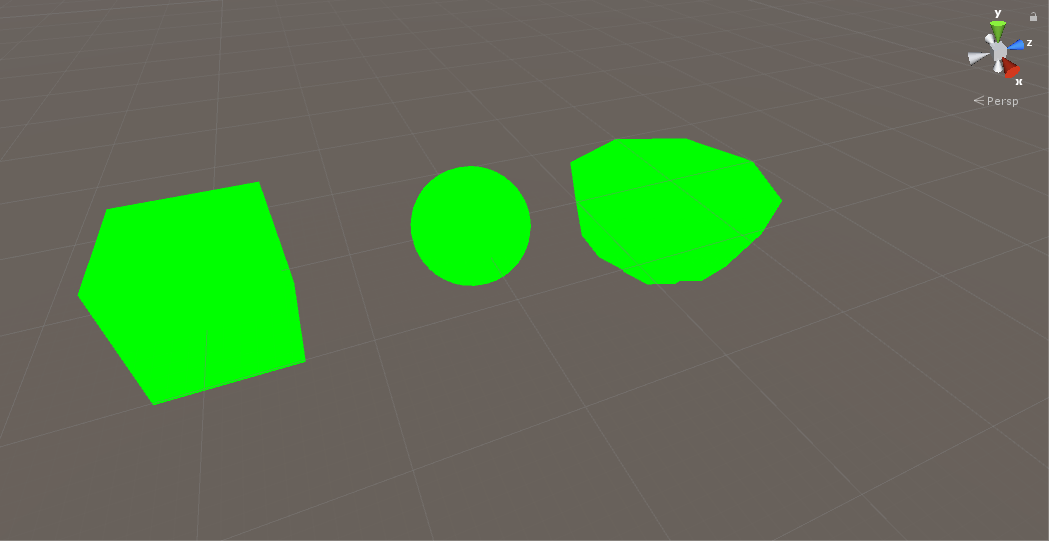name ("display name",type)=default value

Properties{
}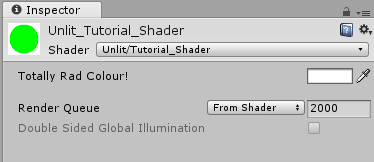CGPROGRAM
#pragma vertex vertexFunction
#pragma fragment fragmentFunction

#include "UnityCG.cginc"

struct a2v{
float4 vertex:POSITION;
float2 uv:TEXCOORD0;
};
struct v2f{
float4 position:SV_POSITION;
float2 uv:TEXCOORD0;
};
//从CG中获取属性
float4 _Color;
v2f vertexFunction(a2v v){
v2f o;
o.position=UnityObjectToClipPos(v.vertex);
return o;
}
fixed4 fragmentFunction(v2f i):SV_TARGET{
return fixed4(0,1,0,1);
}
ENDCG

fixed4 fragmentFunction(v2f i):SV_TARGET{
return _Color;
}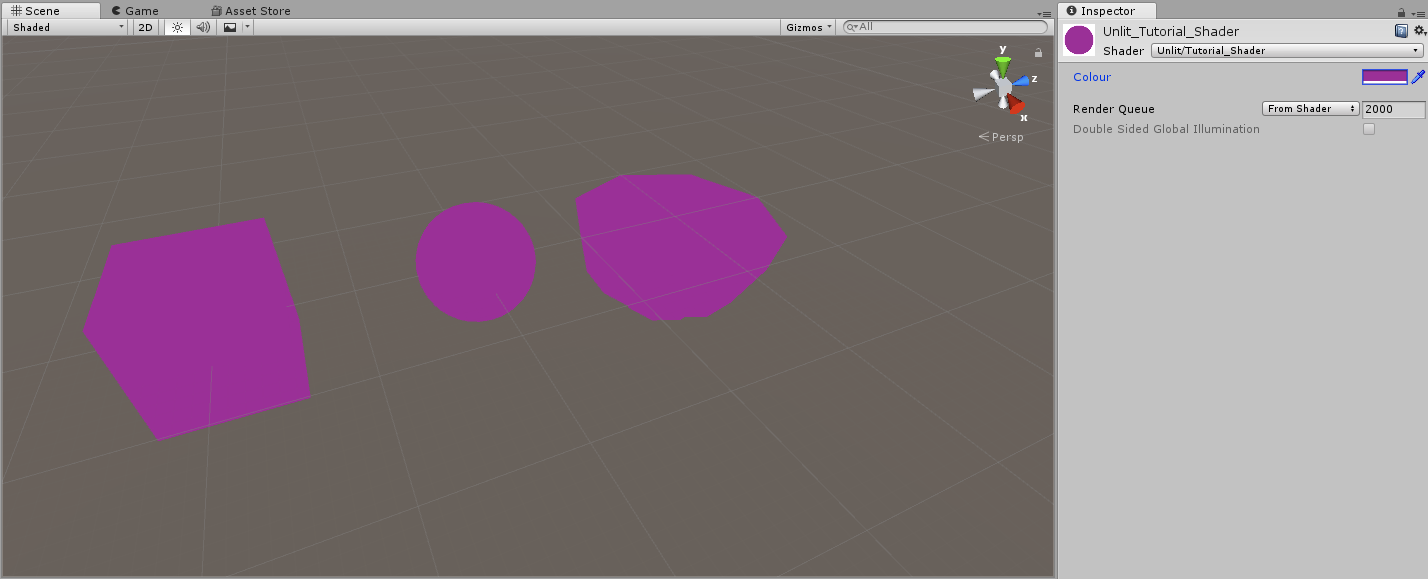Properties{
_Color("_Color",Color)=(1,1,1,1)
_MainTexture("Mian Texture",2D)="white"{}
}

float4 _Color;
sampler2D _MainTexTure;

v2f vertexFunction(a2v v){
v2f o;
o.position=UnityObjectToClipPos(v.vertex);
o.ov=v.uv;
return o;
}

fixed4 fragmentFunction(v2f i):SV_TARGET{
return tex2D(_MainTexture,i.uv);
}

tex2D获取我们想要采样的纹理以及我们想要采样的UV坐标，在这种情况下。我们提供了它的主纹理并给定模型的点，我们可以得到我们想要的颜色，最后返回的是最终的颜色。现在，保存并返回到Unity 的material insepct面板中，选择bowel纹理赋予”Main Texture”“,你会发现，模型发生了改变，尤其是碗的模型看起来尤其像一碗汤。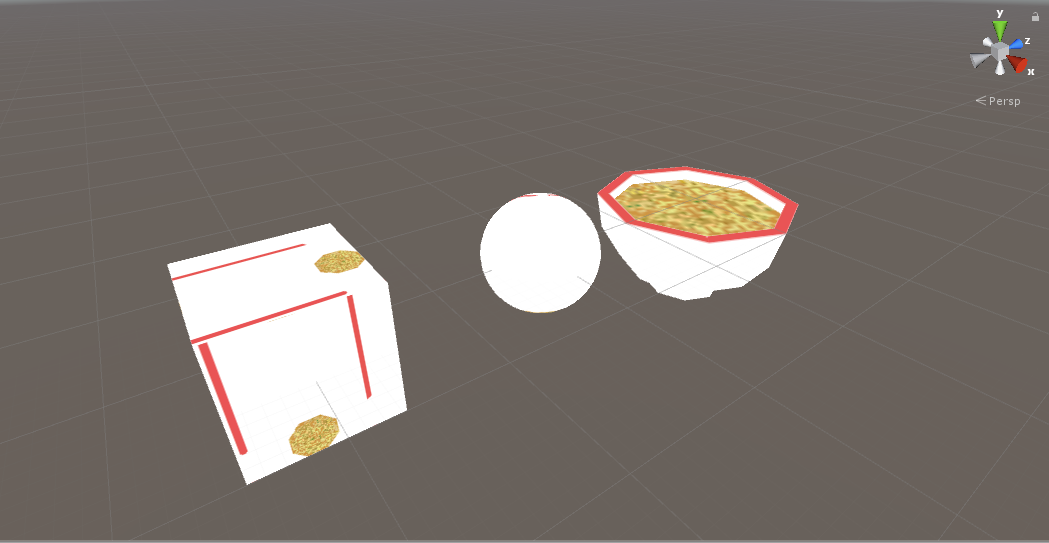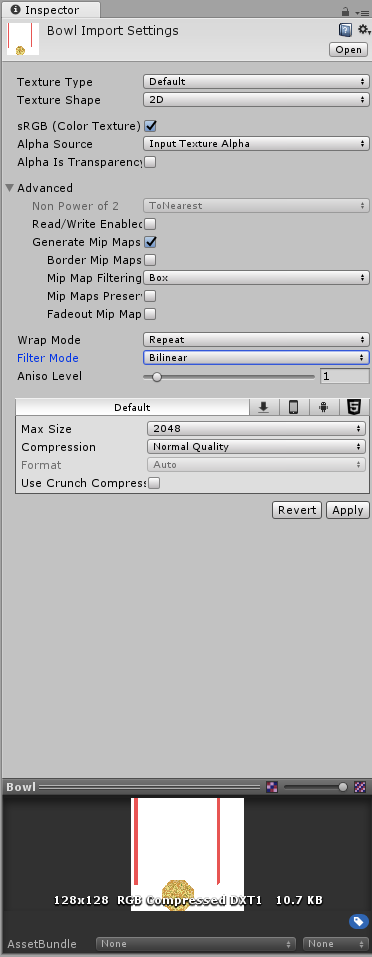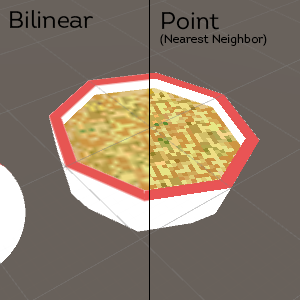Properties{
_Color("Color",Color)=(1,1,1,1)
_MainTexture("Main Texture",2D)="white"{}
_DissolveTexture("Dissolve Texture",2D)="white"{}
_DissolveCutoff("Dissolve Cutoff",Range(0,1)=1
}

float4 _Color;
sampler2D _MainTexture;
sampler2D _DissolveTexture;
float _DissolveCutoff;

fixed4 fragmentFunction(v2f i):SV_TARGET{
float4 textureColor=tex2D(_MainTexture,i.uv);
float4 dissolveColor=tex2D(_DissolveCutoff,i.uv);
return textureColor;
}

fixed4 fragmentFunction(v2f i):SV_TARGET{
float4 textureColor=tex2D(_MainTexture,i.iv);
float4 dissolveColor=tex2D(_DissolveTexture,i.uv);
clip(dissolveColor.rgb-_DissolveCutoff);
return textureColor;
}

clip 函数检查这个给定的值是否小于0.如果小于0，我们将丢弃这个像素并且不绘制它。如果大于0，继续保持像素、正常的渲染，我们的代码的工作方式如下：
1. 对主纹理的颜色进行采样
2. 对纹理颜色进行裁剪采样
3. 从裁剪纹理中减去裁剪值
4. 如果小于0，不进行绘制
5. 否则，返回主纹理采样颜色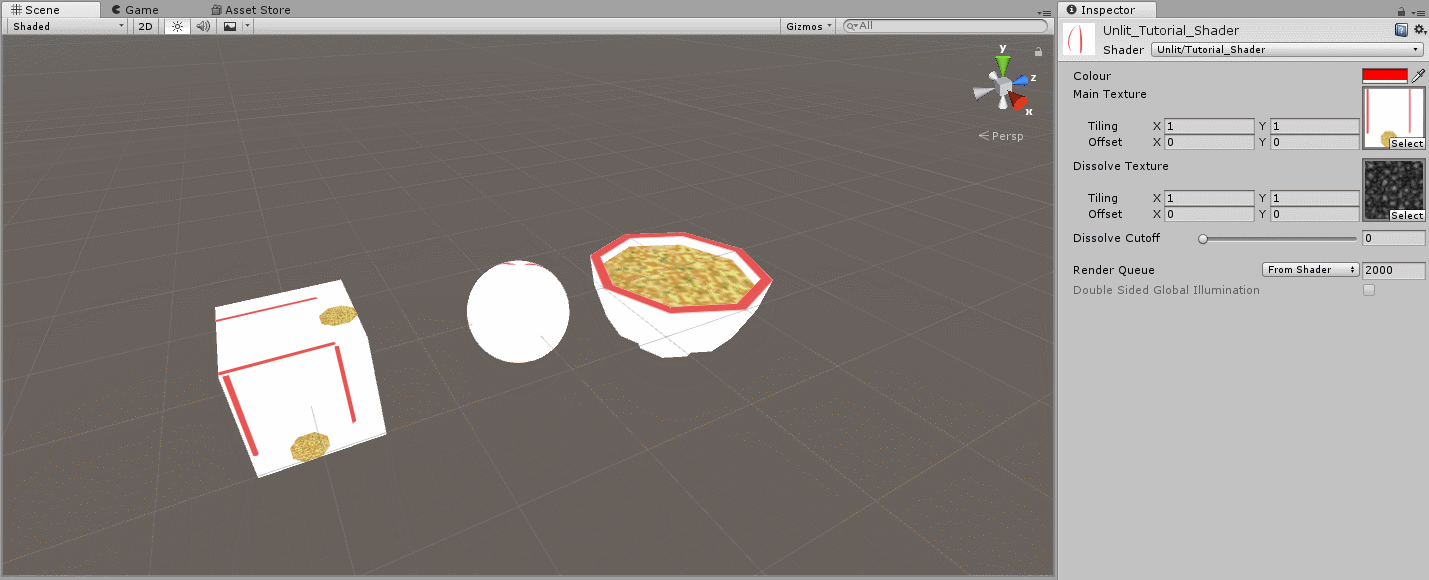Properties{
_Color("Color",Color)=(1,1,1,1)
_MainTexture("Main Texture",2D)="white"{}
_DissolveTexture("Dissolve Texture",2D)="white"{}
_DissolveCuroff("Dissolve Cutoff",Range(0,1))=1
_ExtrudeAmount("Extrue Amount",float)=0
}
...

float4 _Color;
sampler2D _MainTexture;
sampler2D _DissolveTexture;
float _DissolveCutoff;
float _ExtrudeAmount;

struct a2v{
float4 vertex:POSITION;
float2 uv:TEXCOORD0;
float3 normal:NORMAL;
};

v2f vertexFunction(a2v v){
v2f o;
v.vertex.xyz+=v.normal.xyz*_ExtrudeAmount;
o.position=UnityObjectToClipPos(v.vertex);
o.uv=v.uv;
return o;
}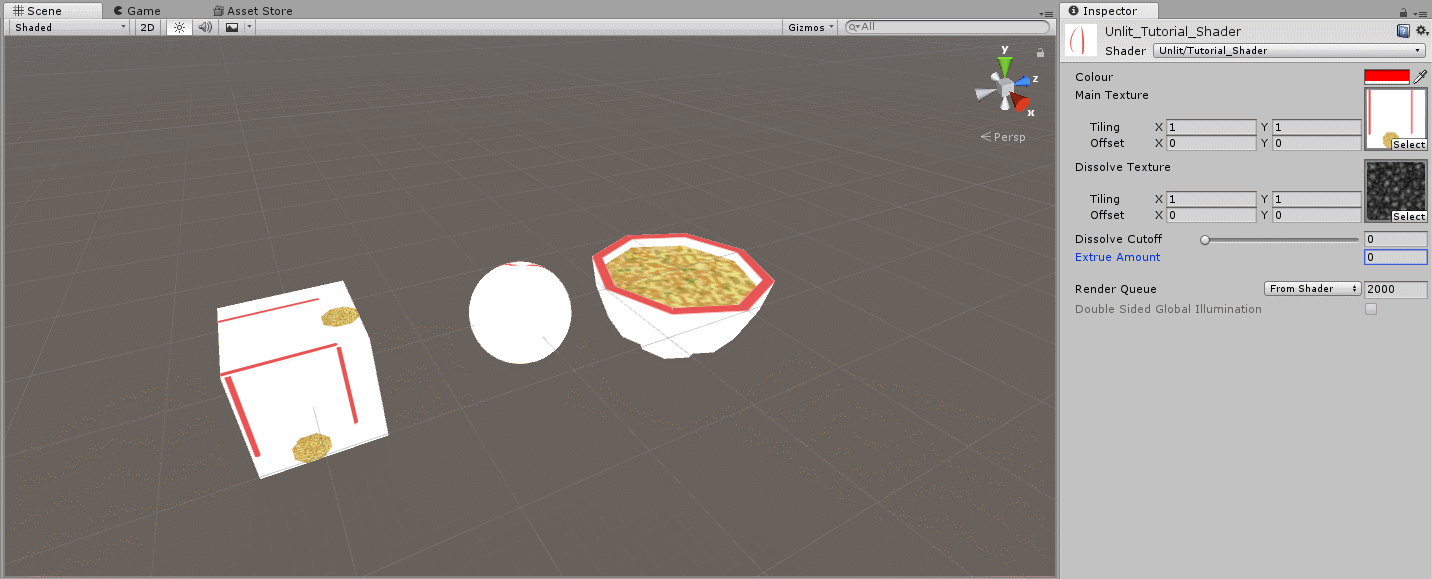v2f vertexFunction(a2v v){
v2f o;
v.vertex.xyz+=v.normal.xyz*_ExtrudeAmount*sin(_Time.y);
o.position=UnityObjectToClipPos(v.vertex);
o.uv=v.uv;
return o;
}

_Time是一个代表时间的变量被包含在UnityCH.cginc中，y值代表秒，确保“Animated Materials” 在场景视图中被勾选，如下：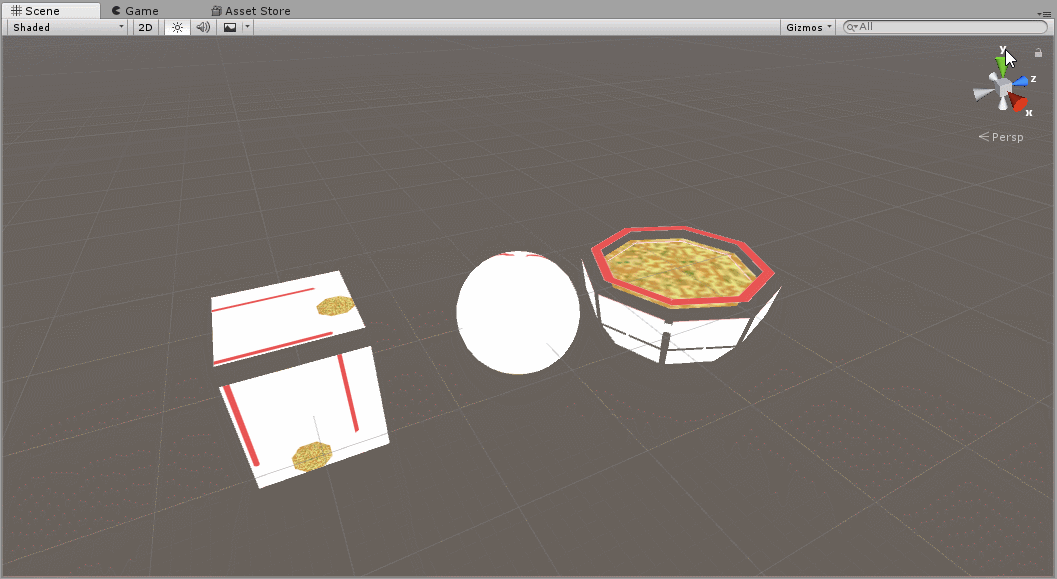Shader "Unlit/Tutorial_Shader"{
Properties{
_Color("Color",Color)=(1,1,1,1)
_MainTexture("Main Texture",2D)="white"{}
_DissolveTexture("Dissolve Texture",2D)="white"{}
_DissolveCutoff("Dissolve Cutoff",Range(0,1))=1
_ExtrudeAmount("Extrue Amount",float)=0
}
Pass{
CGPROGRAM
#pragma vertex vertexFunction
#pragma fragment fragmentFunction
#include "UnityCG.cginc"

struct a2v{
float4 vertex:POSITION;
float2 uv:TEXCOORD0;
float3 normal:NORMAL;
};
struct v2f{
float4 position:SV_POSITION;
flaot2 uv:TEXCOORD0;
};
float4 _Color;
sampler2D _MainTexture;
sampler2D _DissolveTexture;
float _DissolveCutoff;
float _ExtrudeAmount;

v2f vertexFunction(a2v v){
v2f o;
v.vertex.xyz+=v.normal.xyz*_ExtrudeAmount*sin(_Time.y);
o.position=UnityObjectToClipPos(v.vertex);
o.uv=v.uv;
return o;
}

fixed4 fragmentFunction(v2f i):SV_TARGET{
float4 textureColor=tex2D(_MainTexture,i.uv);
float4 dissolveColor=tex2D(_DissolveTexture,i.uv);
clip(dissolveColor.rgb-_DissolveCutoff);
return textureColor;
}
ENDCG
}
}
}

fixed4 fragmentFunction(v2f i):SV_TARGET{
float4 textureColor=tex2D(_MainTexture,i.uv);
float4 dissolveColor=tex2D(_DissolveTexture,i.uv);
clip(dissolveColor.rgb-_DissolveCutoff);
return textureColor*_Color;
}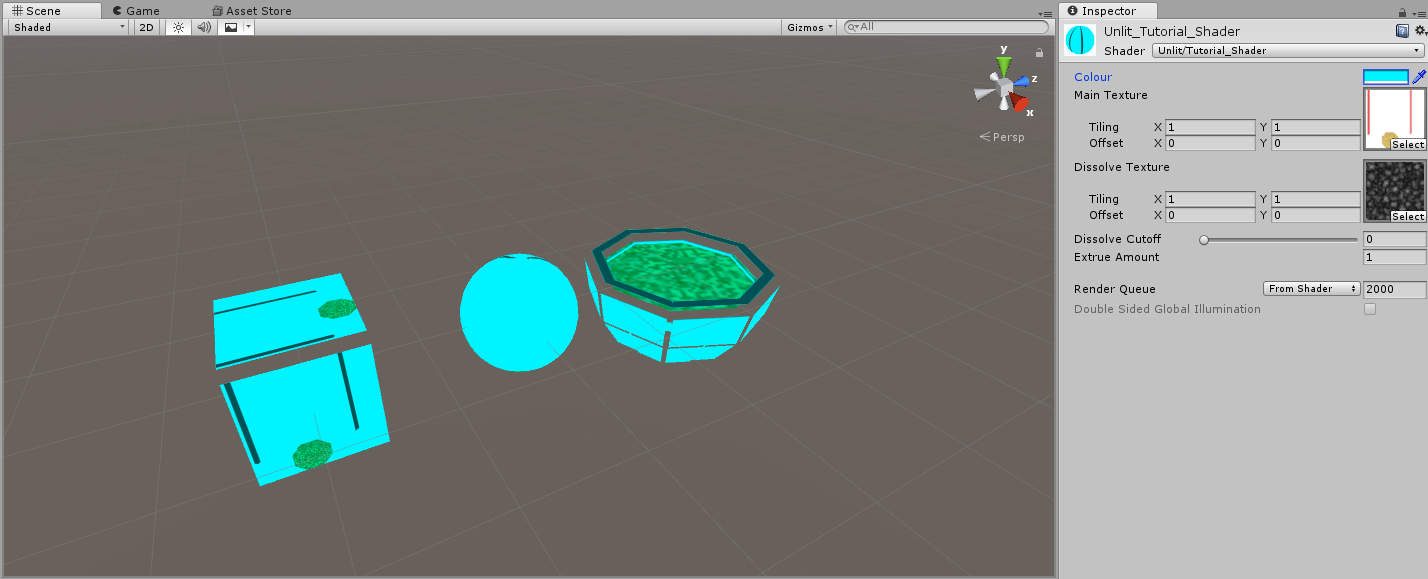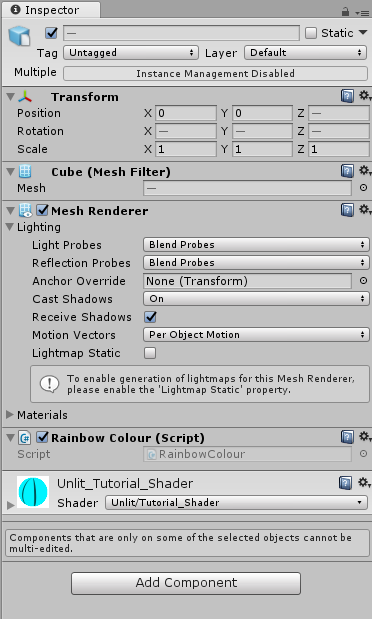using System.Collections;
using System.Collections.Generic;
using UnityEngine;

public class RainbowColor:MonoBehaviour{

Renderer rend;
Material material;

void Start(){

}
void Update(){

}
}

void Start(){
rend=GetComponent<Renderer>();
material=rend.material;
}

void Start(){
rend=GetComponent<Renderer>();
material=rend.material;
material.SetColor("_Color",Color.mangenta);
}### 第八部分：阴影？ 表面着色器？

Shader "Custom/NewSurfaceShader" {
Properties{
_Color("Color",Color)=(1,1,1,1)
_MainTex("Albedo(RGB)",2D)="white"{}
_Glossiness("Smothness",Range(0,1))=0.5
_Metallic("Metallic",Range(0,1))=0.0
}
Tags{"RenderType="Opaque"}
LOD 200

CGPROGRAM
//基于物理着色的光照模型，并且在所有光类型上启用阴影
#pragma surface surf Standard fullforwardshadows
//使用3.0着色器目标，获得更好的光照效果
#pragma target 3.0
sampler2D _MainTex;

struct Input{
float2 uv_MainTex;
};
half _Glossiness;
half _Metrllic;
fixed4 _Color;
//为证着色器添加实例化支持，你需要在材质上检测“启用示例"

UNITY_INSTANCING_CBUFFER_START(Props)

UNITY_INSTANCING_CBUFFER_END

void surf(Input in,inout SurfaceOutputStandard o){
fixed4 c=tex2D(_MainTex,in.uv_MainTex)*_Color;
o.Albedo=c.rgb;
o.Metallic=_Metallic;
o.Smoothness=_Glossiness;
o.Alpha=c.a;
}
ENDCG
}
FallBack "Diffuse"
}

SubShader{
Tags{"RenderType"="Opaque"}
...
}

LOD 200

#pragma surface surf Standard fullforwardshadows

#pragma target 3.0

void surf(Input i,inout SurfaceOutputStandard o){
fixed4 c=tex2D(+mAINtEX,i.uv_MianT)*_Color;
o.Albedo=c.rgb;

o.Metallic=_Metallic;

o.Smoothness=_Glossiness;
o.Alpha=c.a;
}

struct SurfaceOutput{
fixed3 Albedo;
fixed3 Normal;
fixed3 Emission;
half Specular;
fixed Gloss;
fixed Alpha;
}

Okay,讨论一下关于verties把。
standard surface 默认情况下不暴露编辑vertices属性的函数，我们可以手动添加一个。首先，添加pragma并定义一个vertex函数

#pragma surface surf Standard fullforwardshadows vertex:vert

void vert(inout appdata_full v){
v.vertex.xyz+=v.normal.xyz*_ExtrudeAmount*sin(_Time.y;
)
}

#pragma surface surf Standard fullforwardshadows vetex:vert addshadow